Скачать презентацию Newton s Laws — continued Friction Inclined Planes N

13f59a5839d2d7456a081fd571c22539.ppt

• Количество слайдов: 19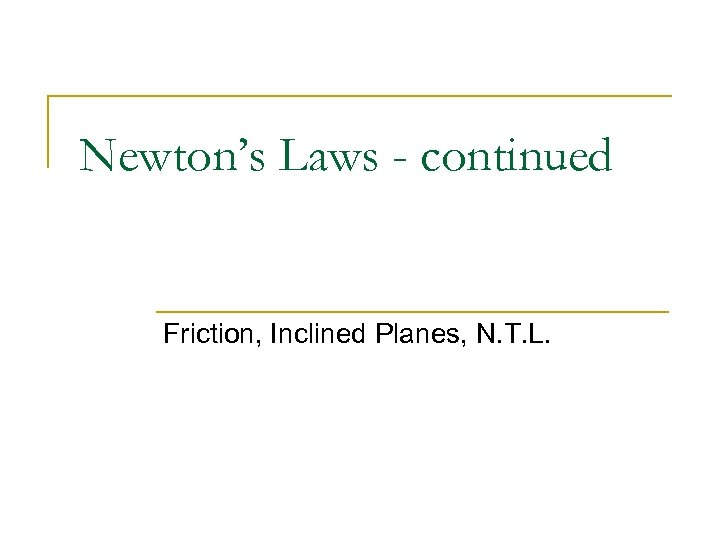Newton’s Laws - continued Friction, Inclined Planes, N. T. L.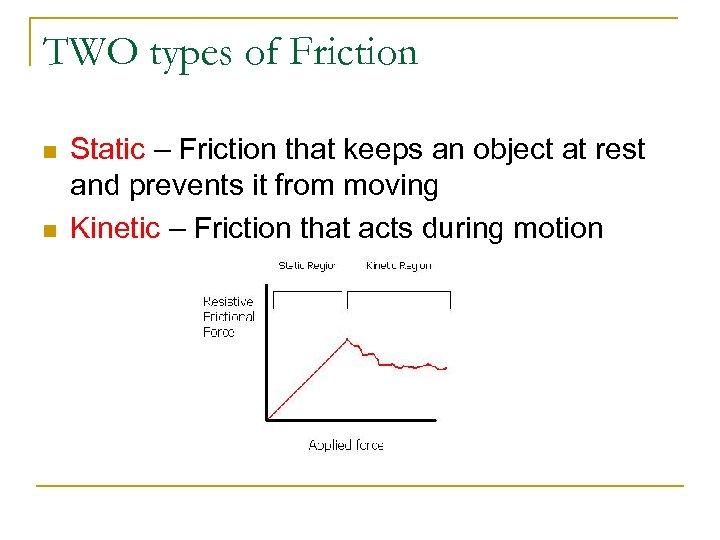TWO types of Friction n n Static – Friction that keeps an object at rest and prevents it from moving Kinetic – Friction that acts during motion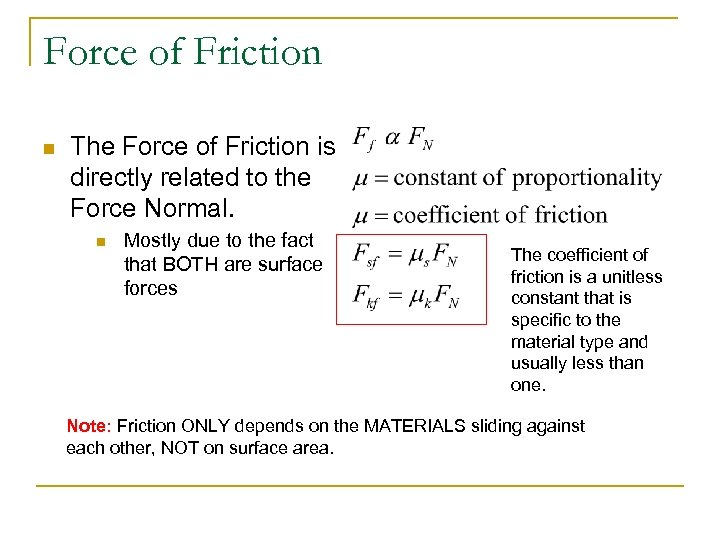Force of Friction n The Force of Friction is directly related to the Force Normal. n Mostly due to the fact that BOTH are surface forces The coefficient of friction is a unitless constant that is specific to the material type and usually less than one. Note: Friction ONLY depends on the MATERIALS sliding against each other, NOT on surface area.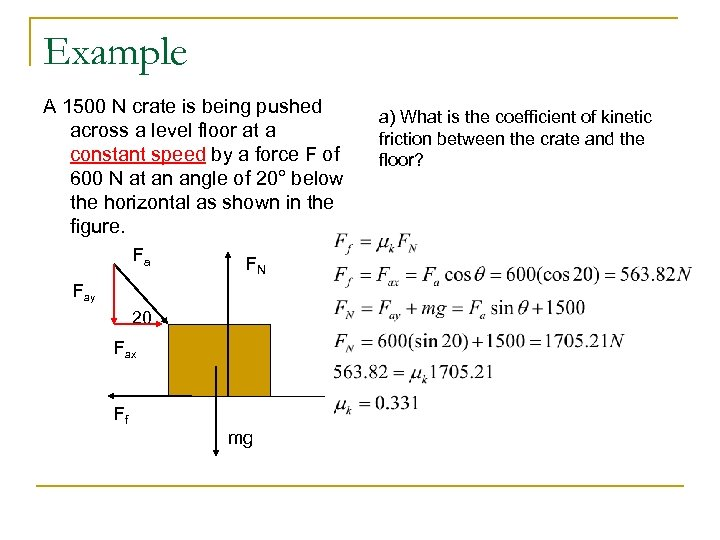Example A 1500 N crate is being pushed across a level floor at a constant speed by a force F of 600 N at an angle of 20° below the horizontal as shown in the figure. Fa FN Fay 20 Fax Ff mg a) What is the coefficient of kinetic friction between the crate and the floor?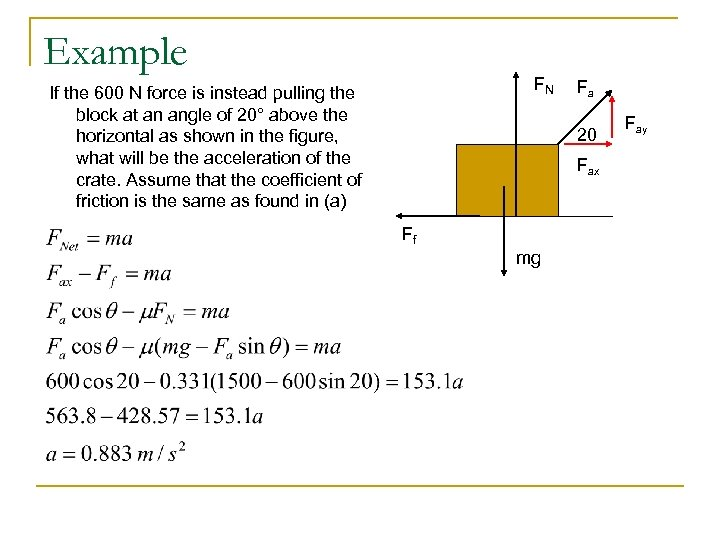Example FN If the 600 N force is instead pulling the block at an angle of 20° above the horizontal as shown in the figure, what will be the acceleration of the crate. Assume that the coefficient of friction is the same as found in (a) Fa 20 Fax Ff mg Fay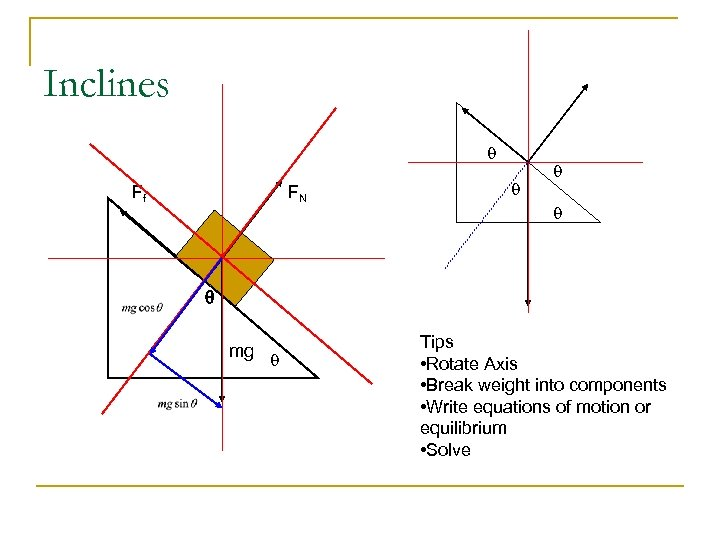Inclines q Ff FN q q mg q Tips • Rotate Axis • Break weight into components • Write equations of motion or equilibrium • Solve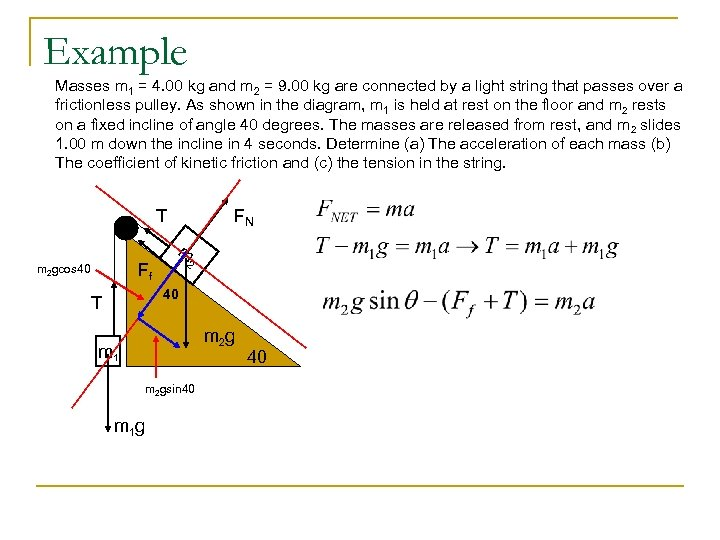Example Masses m 1 = 4. 00 kg and m 2 = 9. 00 kg are connected by a light string that passes over a frictionless pulley. As shown in the diagram, m 1 is held at rest on the floor and m 2 rests on a fixed incline of angle 40 degrees. The masses are released from rest, and m 2 slides 1. 00 m down the incline in 4 seconds. Determine (a) The acceleration of each mass (b) The coefficient of kinetic friction and (c) the tension in the string. T FN m 2 Ff m 2 gcos 40 40 T m 2 g m 1 m 2 gsin 40 m 1 g 40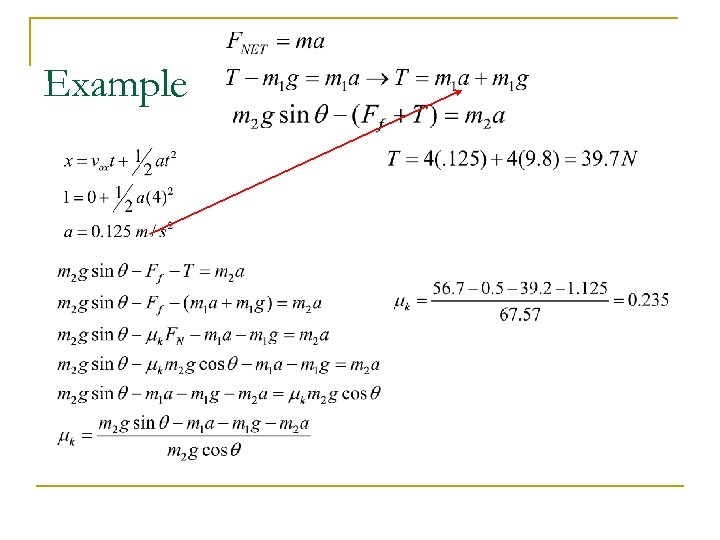Example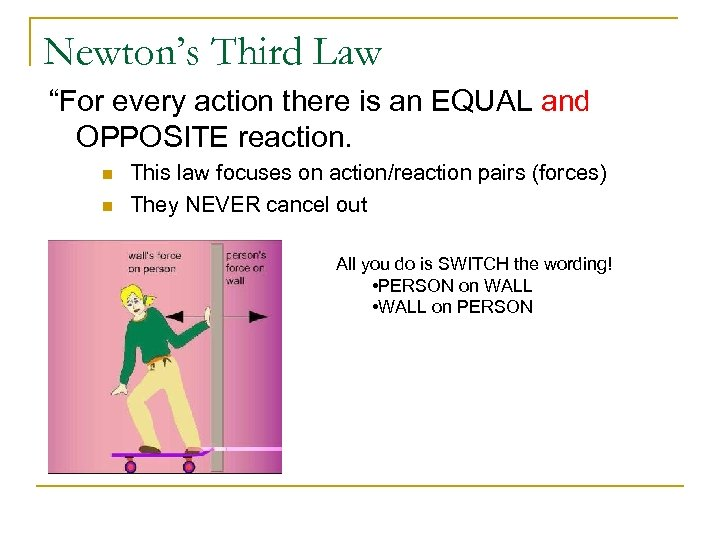Newton’s Third Law “For every action there is an EQUAL and OPPOSITE reaction. n n This law focuses on action/reaction pairs (forces) They NEVER cancel out All you do is SWITCH the wording! • PERSON on WALL • WALL on PERSON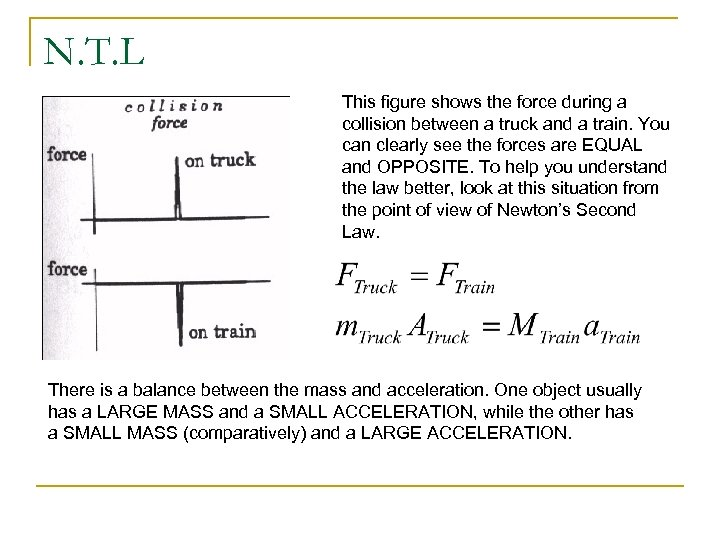N. T. L This figure shows the force during a collision between a truck and a train. You can clearly see the forces are EQUAL and OPPOSITE. To help you understand the law better, look at this situation from the point of view of Newton’s Second Law. There is a balance between the mass and acceleration. One object usually has a LARGE MASS and a SMALL ACCELERATION, while the other has a SMALL MASS (comparatively) and a LARGE ACCELERATION.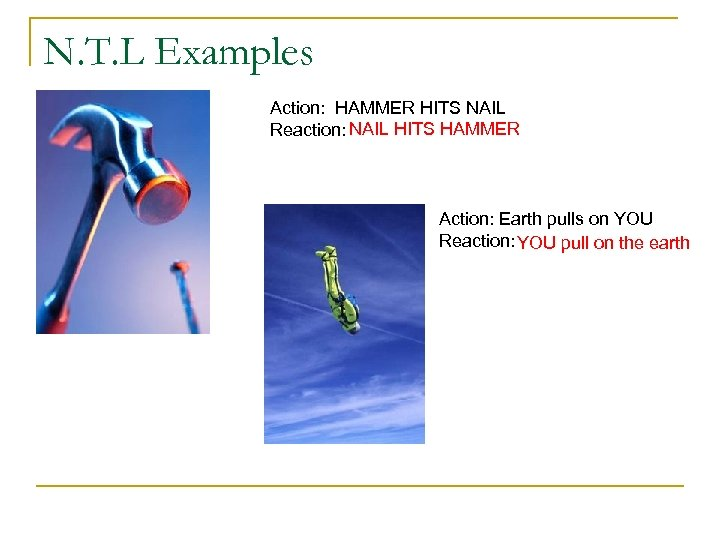N. T. L Examples Action: HAMMER HITS NAIL Reaction: NAIL HITS HAMMER Action: Earth pulls on YOU Reaction: YOU pull on the earth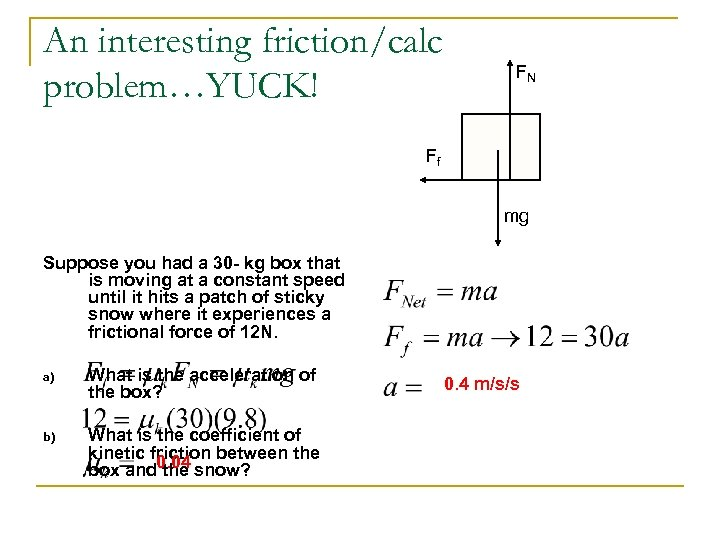An interesting friction/calc problem…YUCK! FN Ff mg Suppose you had a 30 - kg box that is moving at a constant speed until it hits a patch of sticky snow where it experiences a frictional force of 12 N. a) What is the acceleration of the box? b) What is the coefficient of kinetic friction between the box and 0. 04 snow? the 0. 4 m/s/s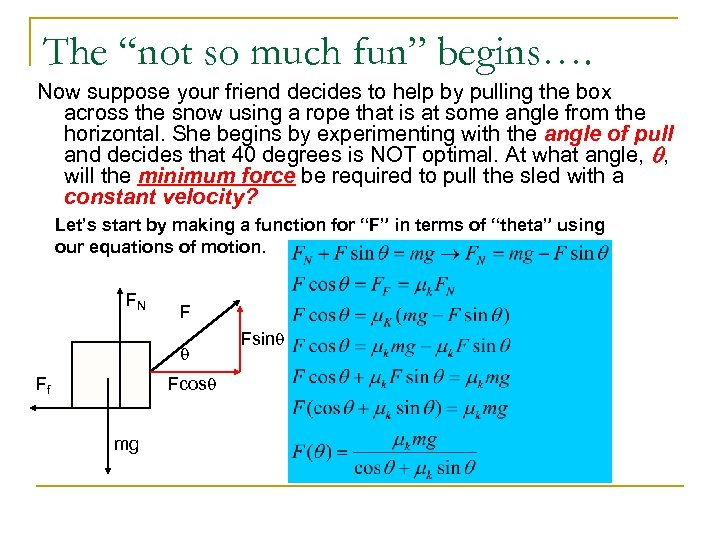The “not so much fun” begins…. Now suppose your friend decides to help by pulling the box across the snow using a rope that is at some angle from the horizontal. She begins by experimenting with the angle of pull and decides that 40 degrees is NOT optimal. At what angle, q, will the minimum force be required to pull the sled with a constant velocity? Let’s start by making a function for “F” in terms of “theta” using our equations of motion. FN F q Fcosq Ff mg Fsinq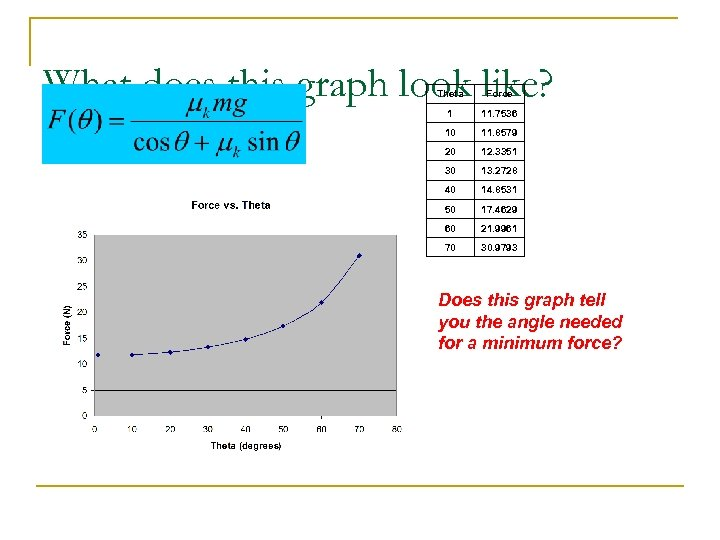What does this graph look like? Theta Force 1 11. 7536 10 11. 8579 20 12. 3351 30 13. 2728 40 14. 8531 50 17. 4629 60 21. 9961 70 30. 9793 Does this graph tell you the angle needed for a minimum force?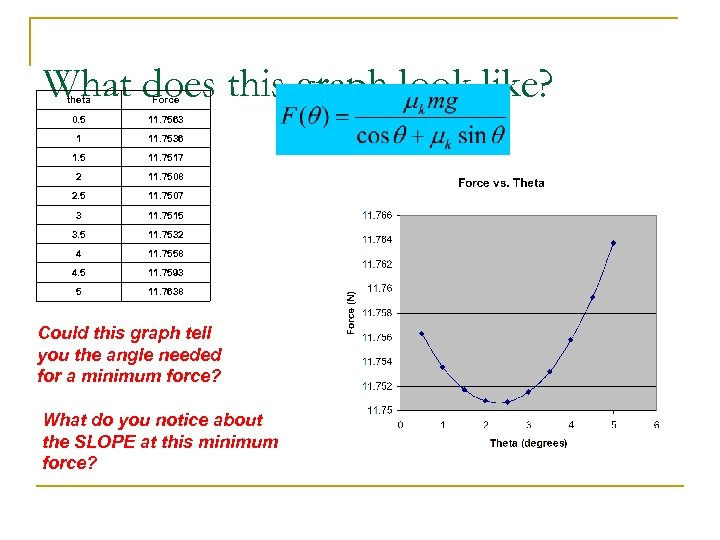What does this graph look like? theta Force 0. 5 11. 7563 1 11. 7536 1. 5 11. 7517 2 11. 7508 2. 5 11. 7507 3 11. 7515 3. 5 11. 7532 4 11. 7558 4. 5 11. 7593 5 11. 7638 Could this graph tell you the angle needed for a minimum force? What do you notice about the SLOPE at this minimum force?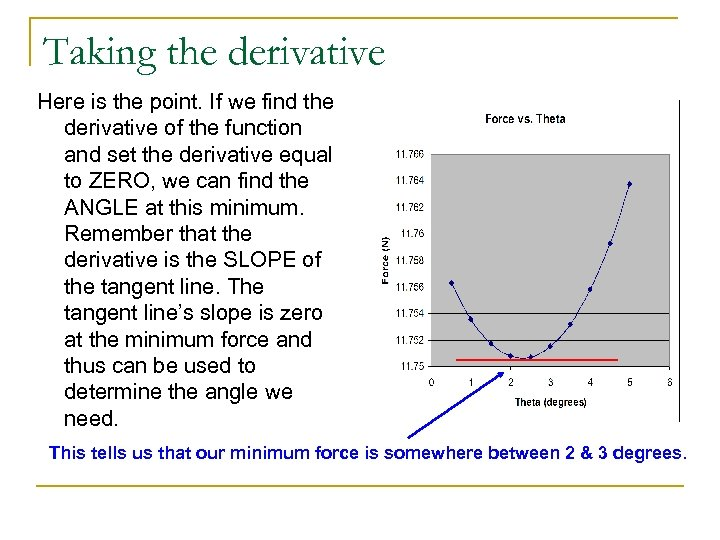Taking the derivative Here is the point. If we find the derivative of the function and set the derivative equal to ZERO, we can find the ANGLE at this minimum. Remember that the derivative is the SLOPE of the tangent line. The tangent line’s slope is zero at the minimum force and thus can be used to determine the angle we need. This tells us that our minimum force is somewhere between 2 & 3 degrees.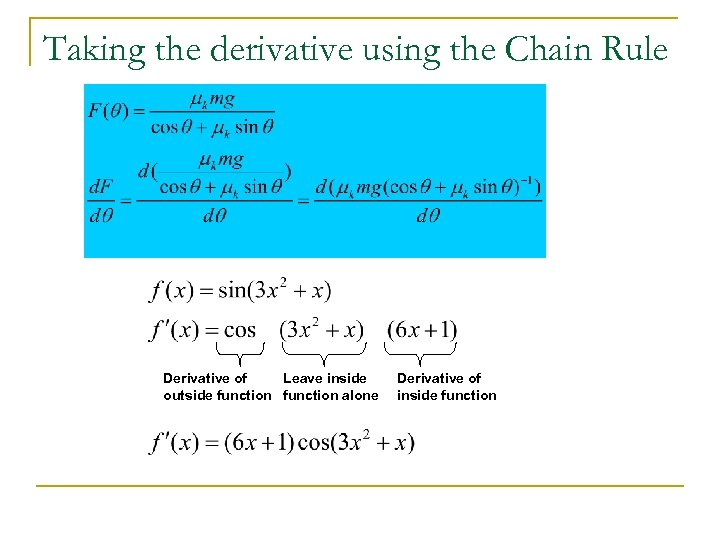Taking the derivative using the Chain Rule Derivative of Leave inside outside function alone Derivative of inside function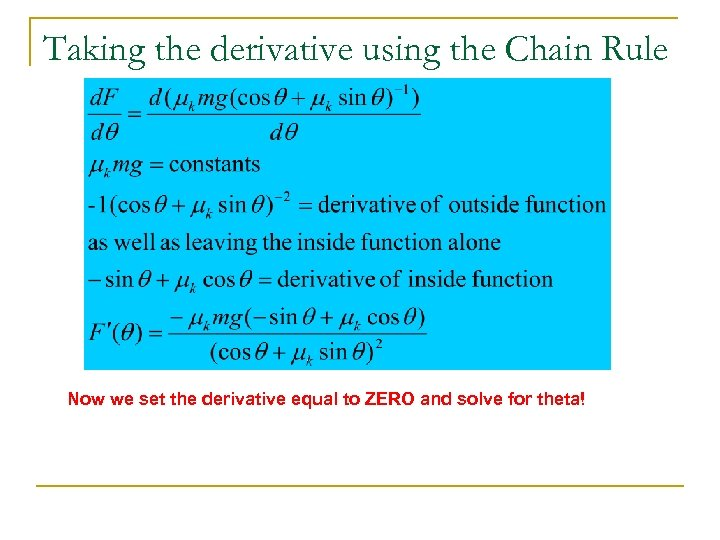Taking the derivative using the Chain Rule Now we set the derivative equal to ZERO and solve for theta!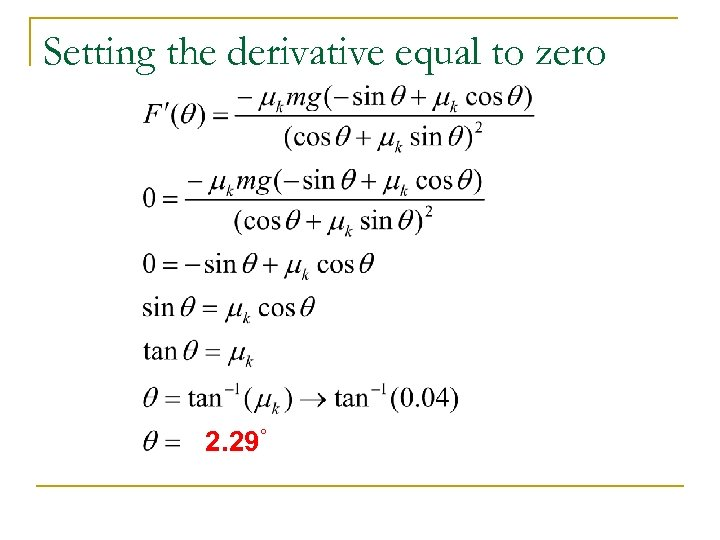Setting the derivative equal to zero 2. 29°